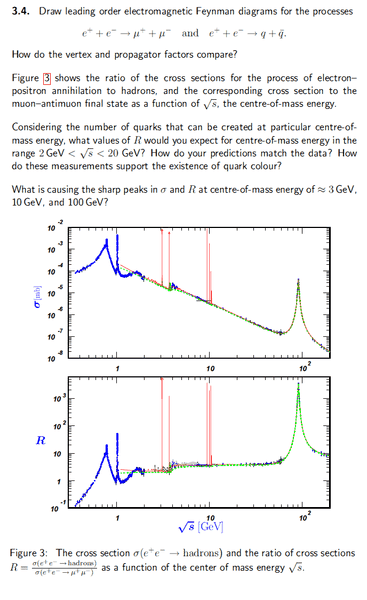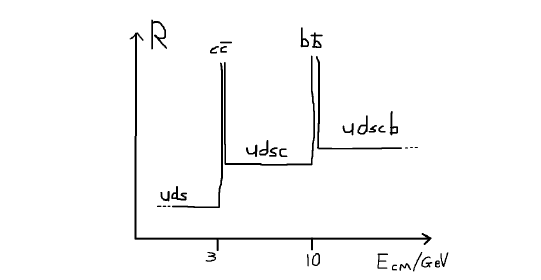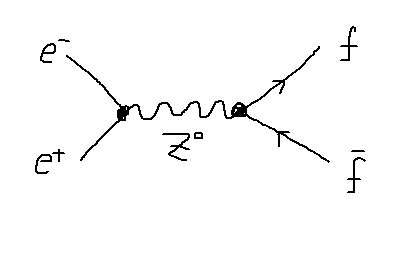# Evidence for Quark Production

## Homework Statement

(a) What are their vertex and propagator factors?
(b) Find the value of R.
(c) Explain the peaks at 3, 10 and 100 GeV.## The Attempt at a Solution

Part (a)
They have the same propagator factor ##\frac{1}{P \cdot P}##.
Vertex factor for muon production is ##(1)(-1)g_{EM}^2 = -g_{EM}^2##.
Vertex factor for hadron production is ##\sum -q^2##.

Part (b)
In the rage of 2GeV to 20GeV, types of quarks produced: u,d,s,c,b.

$$R = 3 \times \frac{e^2\left( -\frac{4}{9} -\frac{1}{9} - \frac{1}{9} - \frac{4}{9} - \frac{1}{9} \right)}{-e^2} = \frac{11}{3}$$

Factor of 3 comes from the 3 colours, R, G and B.

Part (c)
Masses of quarks: u, d ~ ##10^{-3} GeV##, s~ ##0.1GeV##, c~##1 GeV##, b~##4eV##, t~##170GeV##.

Peak at 3GeV comes from production of charmed quarks.
Peak at 10GeV comes from production of bottom quarks.
Peak at 100GeV comes from production of top quarks.

mfb
Mentor
In the rage of 2GeV to 20GeV, types of quarks produced: u,d,s,c,b.
Not all of them are possible over the full range of 2 to 20 GeV.
Peak at 3GeV comes from production of charmed quarks.
Peak at 10GeV comes from production of bottom quarks.
Not the general production, that leads to the flat R you calculated. There is something more special going on at those peaks (related to the quarks).
Peak at 100GeV comes from production of top quarks.
Compare that to the mass of two top quarks. Can that be right?

Not all of them are possible over the full range of 2 to 20 GeV.
Not the general production, that leads to the flat R you calculated. There is something more special going on at those peaks (related to the quarks).
Compare that to the mass of two top quarks. Can that be right?

Mass of quarks d,u,s,c,b are given by 0.005, 0.003, 0.1, 1.2, 4.2 GeV respectively. That's clearly lower than 20GeV, what is restricting their formation?

If it's not the heavier quarks that's forming, I'm guessing it's the formation of hadrons?

You're right in saying that two top quarks will have energy of 340GeV, but can't a combination of top and other quarks be formed?

mfb
Mentor
At 20 GeV all of them are possible, but at 2 GeV that is not true.
If it's not the heavier quarks that's forming, I'm guessing it's the formation of hadrons?
There is a different particle at 90 GeV.

The top quark decays before it hadronizes, and even if it would form hadrons they would be above the top mass. Hadrons do not have an energy significantly above the quark masses - of the order of 1 GeV more is possible, but not 80 GeV.

At 20 GeV all of them are possible, but at 2 GeV that is not true.
There is a different particle at 90 GeV.

The top quark decays before it hadronizes, and even if it would form hadrons they would be above the top mass. Hadrons do not have an energy significantly above the quark masses - of the order of 1 GeV more is possible, but not 80 GeV.

Sorry I should have been more specific. Here are the range for quark production:

uds : 2-3 GeV
udsc: 3-10 GeV
udscb: 10-20 GeVTo summarize, peak at 3GeV corresponds to the J/psi ##(c \bar c)## meson being produced, peak at 10GeV corresponds to the upsilon ##(b \bar b)## meson being produced and peak at 90 GeV corresponds to the ##Z^0## Boson.

But Z meson is produced by coupling to all the standard model fermions.Hadronic: ##f = u, d, s ... ##
Leptonic: ## f = e^-, \mu^-, \tau^-##
Other (invis): ##f = \nu_{e, \mu, \tau}##

None of these have mass 45 GeV. How does the Z boson get its mass of 90 GeV?

Last edited:
mfb
Mentor
Right.

None of these have mass 45 GeV. How does the Z boson get its mass of 90 GeV?
It is an elementary particle with this mass. It is not a bound state of other particles.

•unscientific